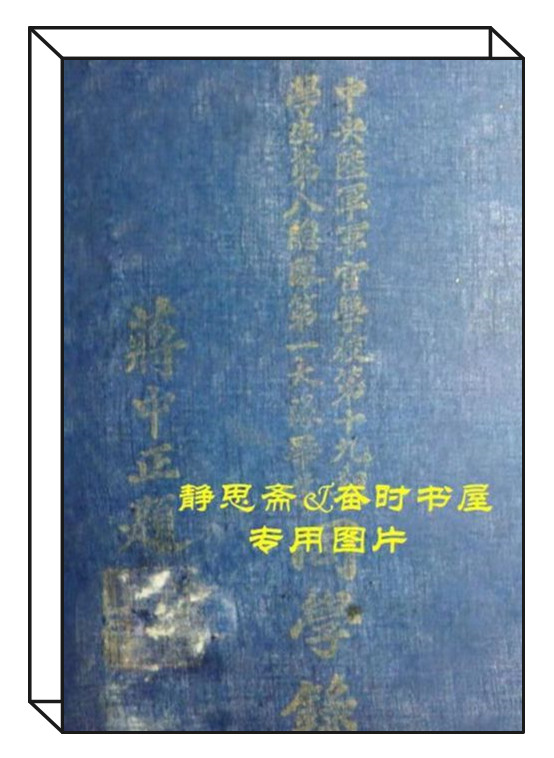•  注 册

# 中央陆军军官学校第十九期第八总队第一大队同学录(黄埔四分校）2022-06-09 08:45:51  来源：静思斋 于岳  点击：说明：本大队毕业于1944年，名录中所载同学282人均有照片收录。静思斋可制作此同学录影印本。

如有后裔需要先辈照片，请与抗日战争纪念网联系。电话：0731-85531328、15116420702(微信同号) ; QQ:2652168198;邮箱：tougao@krzzjn.com;地址：湖南省长沙市岳麓区英才园1片17栋。

第一队：93人

黄中子(广西田东) 彭德球(广西北流) 覃汉(广西上林) 韦建廷(广西武鸣)

林平(广西邕宁) 覃云廷(广西横县) 钟善程(广西容县) 陆济民(广西邕宁)

李杰(广西桂林) 刘家模(广西桂林) 秦毅(广西桂林) 张子倩(安徽徽州)

经宝森(浙江乍浦) 傅融光(四川大邑) 莫桂南(广东高要) 谢树立(湖南衡阳)

艾有桂(贵州独山) 胡光珠(湖北襄阳) 王有槐(贵州独山) 倪奠寰(贵州瓮安)

周礼仪(贵州天柱) 吴青梯(广东大埔) 庾凤山(河南叶县) 梁振亚(河南汲县)

段心照(湖北黄梅) 陈维汉(广东番禺) 张世达(河南博爱) 林成健(湖南武冈)

郑林浦(浙江乐清) 王竹亭(河北获鹿) 何光宗(湖南茶陵) 吴翰(福建永定)

李大良(广西藤县) 韦成循(广西武鸣) 梁兆富(广西融县) 卢蒙彪(广西上林)

王寿廷(广西融县) 朱振明(广西博白) 石永坚(广西义宁) 廖可彦(广西平乐)

班玉瑛(广西邕宁) 蒋作钧(广西恭城) 陈象熙(广西宜山) 朱殿云(广东儋县)

梅亚松(安徽歙县) 李弈标(广西桂平) 龙文骧(湖南安化) 谭觉民(湖南安化)

王星南(河南南阳) 贾铁君(安徽庐江) 张群亮(四川铜梁) 莫荣斌(广西隆安)

江汉清(湖南耒阳) 陈琳璋(湖南湘阴) 李贵(广西南宁) 覃太爵(广西河池)

夏赞陶(湖南邵阳) 卢志修(广西都安) 韦光升(广西那马) 何觉(广西龙津)

林春艳(广西万冈) 李正绪(广西来宾) 林汉民(广西龙津) 黄召明(广东四会)

林茂英(广西都安) 蓝元英(广西上林) 陆清锦(广西武鸣) 吴炳仁(广西藤县)

邢宗发(湖北黄梅) 刘少明(广东南海) 梁子劲(广西怀集) 何德标(湖南永明)

梁雄(广西恭城) 黄文源(广西横县) 萧元星(广西昭平) 刘鸿域(贵州松涛)

曾国儒(广西宾阳) 于东语(广西贺县) 徐达(广西荔浦) 蒋志斌(广西全县)

刘雄俊(广西龙茗) 王喜科(广西藤县) 周尚华(广西灵川) 黄瑞珍(广西龙茗)

赵呈奎(广西恭城) 李家元(广西临桂) 黄汉生(广西容县) 卢寿廷(广西永淳)

覃启荣(广西上林) 周世全(广西平南) 颜乃钧(广西邕宁) 樊任康(广西上林)

章灿元(浙江富阳)

第二队：94人

冯俊德(河南南阳) 覃雄才(广西象县) 陈震寰(安徽霍邱) 朱庄干(山东滋阳)

王树田(湖南长沙) 郑仲琴(广东五华) 任万铭(河南汲县) 林裕友(广西南宁)

陈邦泮(广东文昌) 何定贤(广西武鸣) 周智通(广西融县) 陈智生(广东五华)

张扬(广东龙川) 黄玉俊(广东化县) 温振中(广西容县) 程家声(广西崇善)

李敬武(广东龙门) 谭熙(广西武宣) 黎听上(广西扶南) 覃萃宏(广西上金)

张诚(湖南浏阳) 任厚(河南温县) 莫远文(广西平乐) 高崇麟(辽宁凤城)

王庆善(辽宁凤城) 马德信(辽宁锦县) 周继明(四川叙永) 卢斌(广西来宾)

张凤炜(广西北流) 潘锡禄(广西容县) 罗素明(湖南浏阳) 何浩勋(广西上金)

李德文(湖北建始) 王师(河北清苑) 孙景海(辽宁锦西) 周策(四川铜梁)

刘修年(湖南岳阳) 李镇球(广西贺县) 吴宇宙(广西平南) 于印华(辽宁新民)

吕松(广西陆川) 陈谋(广西容县) 方汉民(广西邕宁) 梁世祖(广西邕宁)

黄启堂(广东中山) 张铭鼐(河北徐水) 杨旭卿(湖南澧县) 卢幼侯(贵州兴义)

孟化然(四川成都) 黄钟麟(广西同正) 朱楷(四川南川) 何云(广西宜山)

朱光明(湖南武冈) 邓名翰(湖南武冈) 梅幼魁(湖南常德) 陈国品(四川新津)

马日新(山东邹县) 林仲春(广西田西) 曾瑞(广西邕宁) 李高月(广西上林)

韩世理(广西上思) 萧玉光(广西龙津) 陶世兴(广西武鸣) 欧庆能(广西来宾)

牧良(安徽南陵) 濮泽宽(安徽南陵) 郭敦盈(江苏镇江) 费明琮(江苏武进)

青柱生(广西南宁) 许世荣(四川南充) 余景诚(四川巴县) 孙超(江苏萧县)

周尚德(广西容县) 凌骥(广东始兴) 陈光元(广东南海) 黄梅(广西百寿)

刘纯(河南息县) 徐克(湖南新化) 张重汉(广西来宾) 王作良(广东郁南)

颜耀(广西南宁) 黄巨卿(广西都安) 崔占先(河南鄢陵) 梁作权(广西平乐)

许士杰(河南汝南) 张镇(河北东光) 江继贤(江西萍乡) 戚连元(山东武城)

覃永珍(广西蒙山) 林如良(贵州毕节) 廖云从(贵州榕江) 周乐群(贵州榕江)

李超建(广西上林) 阳麒广(广西上林)

第三队：95人

班广富(广东钦县) 谢俊生(广西玉林) 张志明(广东梅县) 侯显庭(山东蓬莱)

裴家驹(广东四会) 韦国材(广西思乐) 赵秀盛(广西宾阳) 丁启云(广东兴宁)

叶志伟(广东惠阳) 熊炳根(江西弋阳) 刘武成(广东南海) 黄妙成(广东五华)

何侣章(广东合浦) 陈德超(贵州贵阳) 邹启超(广东五华) 萧慕荣(广东河源)

菅树元(山东阳信) 邓鸿谟(广东茂名) 古求吉(广东五华) 李发漪(江西兴国)

黄醒民(广西邕宁) 覃德卿(广西南宁) 廖凤洲(广东电白) 彭雅儒(广东兴宁)

柯方程(广东茂名) 邓保民(广东茂名) 古晋元(广东五华) 易鹤龄(广西苍梧)

冼雄辉(广东南海) 张振华(四川三台) 李锦新(广东兴宁) 黄湘侯(广西邕宁)

陈同夏(广东遂溪) 李邺(湖南醴陵) 李文富(湖南新田) 王鸿烈(广东电白)

周志达(广东梅县) 韦武俊(广西蒙山) 陆冠华(广西武鸣) 刘永雄(广东廉江)

吴孝钿(江西南康) 何世标(广东始兴) 陈鸿高(广东兴宁) 梁伟南(广东化县)

李堃(广西融县) 李钧庆(广东五华) 陈汉章(广东灵山) 黄乐伍(广西贵县)

何美才(广西藤县) 张雪生(广西柳城) 甘克武(广西容县) 张润进(广东大埔)

刘蕴璋(广东梅县) 李炳霖(广东兴宁) 毕炳燊(广东花县) 李宗橿(广东阳春)

刘锡(广东梅县) 叶荫(广东兴宁) 蒋征(广西玉林) 谭光辉(广西邕宁)

刘森林(四川广汉) 王奠球(广东增城) 谢华海(广东合浦) 王润榕(广东电白)

罗总登(广东大埔) 李劲夫(广东合浦) 罗瑞明(广西左县) 黎壬初(广东灵山)

邓焕辉(广东茂名) 成俊志(山东桓山) 叶福甫(广东合浦) 车汉鹏(广东茂名)

谢金水(广东大埔) 李祖杰(广西贵县) 黄自光(广东新会) 杜铨(四川叙府)

陈戈荣(广西陆川) 刘汉平(广东兴宁) 杨本礼(贵州天柱) 何忠权(广东防城)

卢英明(广西来宾) 李湘(广东新会) 唐济丰(广东信宜) 陈士锋(广东化县)

林华(广东茂名) 邹秉权(广东五华) 谢佯僧(江西遂川) 杨慕尧(广东兴宁)

张有华(广东五华) 杨精吉(四川永川) 冯利清(广东定安) 陈善汉(广东琼山)

吴谈锦(广东定安) 韩惠丹(广东文昌) 胡育彬(广东兴宁)中文域名：www.抗日战争纪念网.com　主办单位：长沙市抗战文化研究会　技术支持：刘庆为